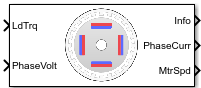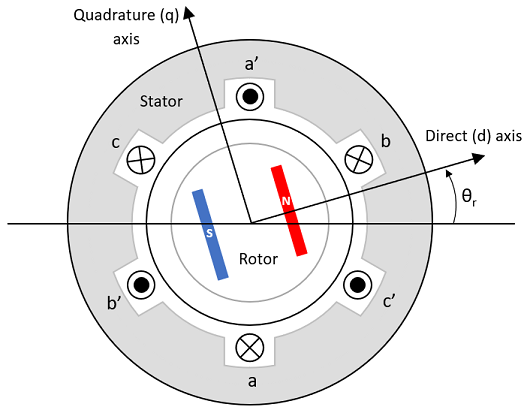# Interior PMSM

Three-phase interior permanent magnet synchronous motor with sinusoidal back electromotive force

• Library:
• Powertrain Blockset / Propulsion / Electric Motors and Inverters

Motor Control Blockset / Electrical Systems / Motors

•## Description

The Interior PMSM block implements a three-phase interior permanent magnet synchronous motor (PMSM) with sinusoidal back electromotive force. The block uses the three-phase input voltages to regulate the individual phase currents, allowing control of the motor torque or speed.

By default, the block sets the Simulation type parameter to `Continuous` to use a continuous sample time during simulation. If you want to generate code for fixed-step double- and single-precision targets, considering setting the parameter to `Discrete`. Then specify a Sample Time, Ts parameter.

On the Parameters tab, if you select `Back-emf`, the block implements this equation to calculate the permanent flux linkage constant.

`${\lambda }_{pm}=\frac{1}{\sqrt{3}}\cdot \frac{{K}_{e}}{1000P}\cdot \frac{60}{2\pi }$`

### Motor Construction

This figure shows the motor construction with a single pole pair on the motor.The motor magnetic field due to the permanent magnets creates a sinusoidal rate of change of flux with motor angle.

For the axes convention, the a-phase and permanent magnet fluxes are aligned when motor angle θr is zero.

### Three-Phase Sinusoidal Model Electrical System

The block implements these equations, expressed in the motor flux reference frame (dq frame). All quantities in the motor reference frame are referred to the stator.

`$\begin{array}{l}{\omega }_{e}=P{\omega }_{m}\\ \frac{d}{dt}{i}_{d}=\frac{1}{{L}_{d}}{v}_{d}-\frac{R}{{L}_{d}}{i}_{d}+\frac{{L}_{q}}{{L}_{d}}P{\omega }_{m}{i}_{q}\end{array}$`

`$\frac{d}{dt}{i}_{q}=\frac{1}{{L}_{q}}{v}_{q}-\frac{R}{{L}_{q}}{i}_{q}-\frac{{L}_{d}}{{L}_{q}}P{\omega }_{m}{i}_{d}-\frac{{\lambda }_{pm}P{\omega }_{m}}{{L}_{q}}$`

`${T}_{e}=1.5P\left[{\lambda }_{pm}{i}_{q}+\left({L}_{d}-{L}_{q}\right){i}_{d}{i}_{q}\right]$`

The Lq and Ld inductances represent the relation between the phase inductance and the motor position due to the saliency of the motor.

The equations use these variables.

 Lq, Ld q- and d-axis inductances (H) R Resistance of the stator windings (ohm) iq, id q- and d-axis currents (A) vq, vd q- and d-axis voltages (V) ωm Angular mechanical velocity of the motor (rad/s) ωe Angular electrical velocity of the motor (rad/s) λpm Permanent flux linkage constant (Wb) Ke Back electromotive force (EMF) (Vpk_LL/krpm, where Vpk_LL is the peak voltage line-to-line measurement) P Number of pole pairs Te Electromagnetic torque (Nm) Θe Electrical angle (rad)

### Mechanical System

The motor angular velocity is given by:

`$\begin{array}{c}\frac{d}{dt}{\omega }_{m}=\frac{1}{J}\left({T}_{e}-{T}_{f}-F{\omega }_{m}-{T}_{m}\right)\\ \frac{d{\theta }_{m}}{dt}={\omega }_{m}\end{array}$`

The equations use these variables.

 J Combined inertia of motor and load (kgm^2) F Combined viscous friction of motor and load (N·m/(rad/s)) θm Motor mechanical angular position (rad) Tm Motor shaft torque (Nm) Te Electromagnetic torque (Nm) Tf Motor shaft static friction torque (Nm) ωm Angular mechanical velocity of the motor (rad/s)

### Power Accounting

For the power accounting, the block implements these equations.

Bus Signal DescriptionVariableEquations

`PwrInfo`

`PwrTrnsfrd` — Power transferred between blocks

• Positive signals indicate flow into block

• Negative signals indicate flow out of block

`PwrMtr`

Mechanical power

Pmot

`PwrBus`

Electrical power

Pbus

`PwrNotTrnsfrd` — Power crossing the block boundary, but not transferred

• Positive signals indicate an input

• Negative signals indicate a loss

`PwrElecLoss`

Resistive power loss

Pelec

`PwrMechLoss`

Mechanical power loss

Pmech

When Port Configuration is set to `Torque`:

When Port Configuration is set to `Speed`:

`PwrStored` — Stored energy rate of change

• Positive signals indicate an increase

• Negative signals indicate a decrease

`PwrMtrStored`

Stored motor power

Pstr

The equations use these variables.

 Rs Stator resistance (ohm) ia, ib, ic Stator phase a, b, and c current (A) isq, isd Stator q- and d-axis currents (A) van, vbn, vcn Stator phase a, b, and c voltage (V) ωm Angular mechanical velocity of the rotor (rad/s) F Combined motor and load viscous damping (N·m/(rad/s)) Te Electromagnetic torque (Nm) Tf Combined motor and load friction torque (Nm)

### Amplitude invariant dq transformation

The block uses these equations to implement amplitude invariant dq transformation to ensure that the dq and three phase amplitudes are equal.

The equations use these variables.

 Θda dq stator electrical angle with respect to the rotor a-axis (rad) vsq, vsd Stator q- and d-axis voltages (V) isq, isd Stator q- and d-axis currents (A) va, vb, vc Stator voltage phases a, b, c (V) ia, ib, ic Stator currents phases a, b, c (A)

## Ports

### Input

expand all

Load torque on the motor shaft, Tm, in N·m.

#### Dependencies

To create this port, select `Torque` for the Port Configuration parameter.

Angular velocity of the motor, ωm, in rad/s.

#### Dependencies

To create this port, select `Speed` for the Port Configuration parameter.

Stator terminal voltages, Va, Vb, and Vc, in V.

#### Dependencies

To create this port, select `Speed` or `Torque` for the Port Configuration parameter.

### Output

expand all

The bus signal contains these block calculations.

Signal DescriptionVariableUnits

`IaStator`

Stator phase current A

ia

A

`IbStator`

Stator phase current B

ib

A

`IcStator`

Stator phase current C

ic

A

`IdSync`

Direct axis current

id

A

`IqSync`

iq

A

`VdSync`

Direct axis voltage

vd

V

`VqSync`

vq

V

`MtrSpd`

Angular mechanical velocity of the motor

ωm

`MtrPos`

Motor mechanical angular position

θm

`MtrTrq`

Electromagnetic torque

Te

N·m

`PwrInfo`

`PwrTrnsfrd`

`PwrMtr`

Mechanical power

Pmot

W
`PwrBus`

Electrical power

Pbus

W

`PwrNotTrnsfrd`

`PwrElecLoss`

Resistive power loss

Pelec

W
`PwrMechLoss`

Mechanical power loss

Pmech

W

`PwrStored`

`PwrMtrStored`

Stored motor power

Pstr

W

Phase a, b, c current, ia, ib, and ic, in A.

Motor torque, Tmtr, in N·m.

#### Dependencies

To create this port, select `Speed` for the Mechanical input configuration parameter.

Angular speed of the motor, ωmtr, in rad/s.

#### Dependencies

To create this port, select `Torque` for the Mechanical input configuration parameter.

## Parameters

expand all

Block Options

This table summarizes the port configurations.

Port ConfigurationCreates Input PortCreates Output Port

`Torque`

`LdTrq`

`MtrSpd`

`Speed`

`Spd`

`MtrTrq`

By default, the block uses a continuous sample time during simulation. If you want to generate code for single-precision targets, considering setting the parameter to `Discrete`.

#### Dependencies

Setting Simulation type to `Discrete` creates the Sample Time, Ts parameter.

Integration sample time for discrete simulation, in s.

#### Dependencies

Setting Simulation type to `Discrete` creates the Sample Time, Ts parameter.

Enter the path to the motor parameter ".m" or ".mat" file that you saved using the Motor Control Blockset™ parameter estimation tool. You can also click the button to navigate and select the ".m" or ".mat" file, and update File parameter with the file name and path. For details related to the motor parameter estimation process, see Estimate PMSM Parameters Using Recommended Hardware.

• - Click this button to read the estimated motor parameters from the ".m" or ".mat" file (indicated by the File parameter) and load them to the motor block.

• - Click this button to read the motor parameters from the motor block and save them into a ".m" or ".mat" file (with a file name and location that you specify in the File parameter).

Note

Before you click button, ensure that the target file name in the File parameter has either ".m" or ".mat" extension. If you use any other file extension, the block displays an error message.

Parameters

Motor pole pairs, P.

Stator phase resistance per phase, Rs, in ohm.

Stator d-axis and q-axis inductance, Ld, Lq, in H.

Permanent flux linkage constant, λpm, in Wb.

Back electromotive force, EMF, Ke, in Vpk_LL/krpm. Vpk_LL is the peak voltage line-to-line measurement.

To calculate the permanent flux linkage constant, the block implements this equation.

`${\lambda }_{pm}=\frac{1}{\sqrt{3}}\cdot \frac{{K}_{e}}{1000P}\cdot \frac{60}{2\pi }$`

Mechanical properties of the motor:

• Inertia, J, in kg.m^2

• Viscous damping, F, in N·m/(rad/s)

• Static friction, Tf, in N·m

#### Dependencies

To enable this parameter, select the `Torque` configuration parameter.

Initial Values

Initial q- and d-axis currents, iq, id, in A.

Initial motor angular position, θm0, in rad.

Initial angular velocity of the motor, ωm0, in rad/s.

#### Dependencies

To enable this parameter, select the `Torque` configuration parameter.

 Kundur, P. Power System Stability and Control. New York, NY: McGraw Hill, 1993.

 Anderson, P. M. Analysis of Faulted Power Systems. Hoboken, NJ: Wiley-IEEE Press, 1995.